# CHSL (10+2) Model Papers Mock Test -4 Arithmetic

## CHSL (10+2) Model Papers Mock Test -4 Arithmetic

CHSL (10+2) Model Papers Mock Test -4 Arithmetic

1. The value of (256)0.16 × (256)0.09 is :

(a) 256.25
(b) 64
(c) 16
(d) 4

2. Simplify :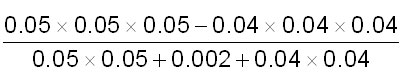3.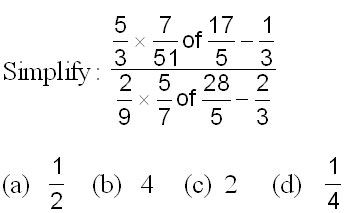4. 1 – [5 – {2 + (–5 + 6 – 2) 2}] is equal to :

(a) –4
(b) 2
(c) 0
(d) –2

5
.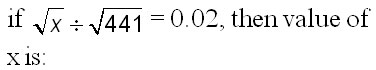(a) 1.64
(b) 2.64
(c) 1.764
(d) 0.1764

6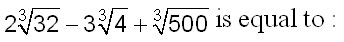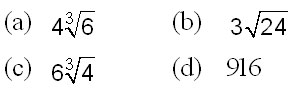7.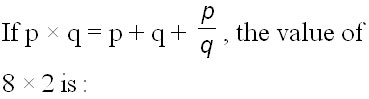(a) 6
(b) 10
(c) 14
(d) 16

8. The square root of :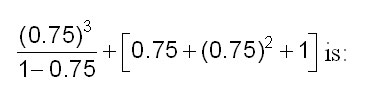(a) 4
(b) 3
(c) 2
(d) 1

9. Find the value of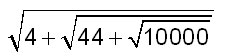(a) 12
(b) 8
(c) 4
(d) –4

10. If 3x + 8 = 272x + 1, the value of x is :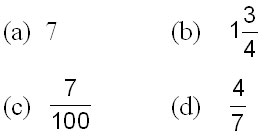11. is equal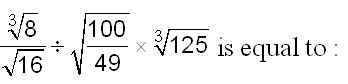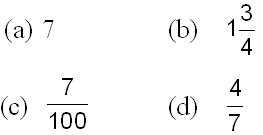12. If log102 = 0.3010 and log107 = 0.8451, then the value of log102.8 is :

(a) 0.4471
(b) 1.4471
(c) 2.4471
(d) 1.4471

13. Which of the following fractions is the smallest?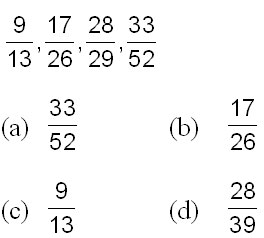14. (49)15 – 1 is exactly divisible by:

(a) 50
(b) 51
(c) 29
(d) 8

15.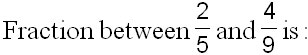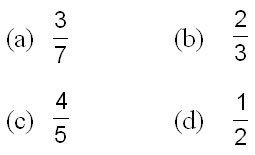16.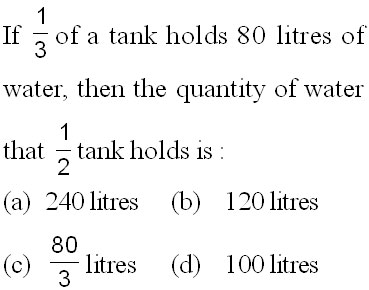17. The LCM of two numbers is 495 and their HCF is 5. If the sum of numbers is 100, then their difference is :

(a) 10
(b) 46
(c) 70
(d) 90

18. LCM of two numbers is 225 and their HCF is 5. If one number is 25, the other number will be :

(a) 5
(b) 25
(c) 45
(d) 225

19.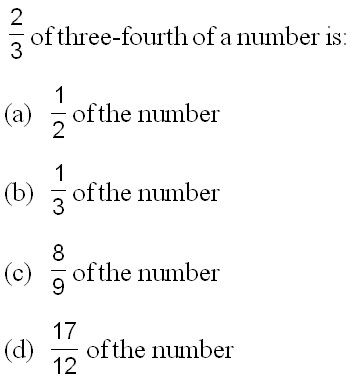20. If we write 45 as sum of four numbers so that when 2 is added to first number, 2 subtracted from second number, third multiplied by 2 and fourth divided by 2, we get the same result, then the four numbers are:

(a) 1, 8, 15, 21
(b) 8, 12, 5, 20
(c) 8, 12, 10, 15
(d) 2, 12, 5, 26

21. Four numbers are in the ratio 1 : 2 : 3 : 4. Their sum is 16. The sum of the first and fourth number is equal to :

(a) 5
(b) 8
(c) 10
(d) 80

22.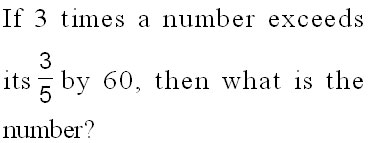(a) 25
(b) 35
(c) 45
(d) 60

23.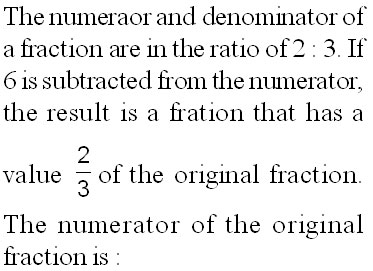(a) 6
(b) 18
(c) 27
(d) 36

24. The sum of three consecutive odd natural numbers is 147. Then, the middle number is :

(a) 47
(b) 48
(c) 49
(d) 51

25. If 5432*2 is divisible by 9, then the digit in place of * is :

(a) 0
(b) 1
(c) 6
(d) 9

26. By which smallest number 1323 must be multiplied, so that it becomes a perfect cube?

(a) 2
(b) 3
(c) 5
(d) 7

27. The least number, which when divided by 12, 15, 20 or 54 leaves a remainder of 4 in each case, is :

(a) 450
(b) 454
(c) 540
(d) 544

28.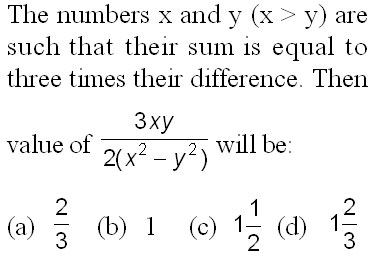29. Four bells ring at intervals of 4, 6, 8 and 14 seconds. They start ringing simultaneously at 12.00 O’clock. At what time will they again ring simultaneously ?

(a) 12 hrs. 2 min. 48 sec.
(b) 12 hrs. 3 min.
(c) 12 hrs. 3 min. 20 sec.
(d) 12 hrs. 3 min. 44 sec.

30.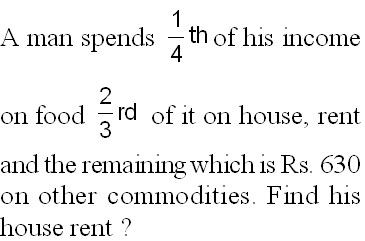(a) Rs. 5040
(b) Rs. 3520
(c) Rs. 4890
(d) Rs. 4458

31. A number is doubled and 9 is added. If the resultant is trebled, it becomes 75. What is that number?

(a) 6
(b) 3.5
(c) 8
(d) None of these

32. A number when divided by 899 gives a remainder 63. If the same number is divided by 29, the remainder will be:

(a) 10
(b) 5
(c) 4
(d) 2

33.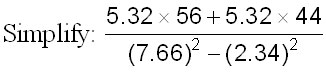(a) 7.2
(b) 8.5
(c) 10
(d) 12

34. Which of the following is the biggest?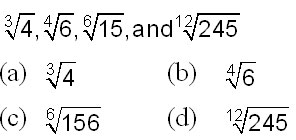35. If 120 is 20% of a number, then 120% of that number will be:

(a) 20
(b) 120
(c) 480
(d) 720

36. If 8% of x is the same as 4% of y, then 20% of x is the same as:

(a) 10% of y
(b) 16% of y
(c) 80% of y
(d) 50% of y

37. If x is less than y by 25% then y exceed x by: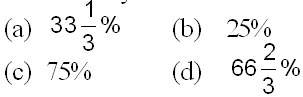38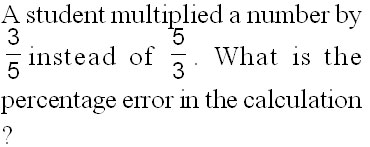(a) 44%
(b) 34%
(c) 54%
(d) 64%

39.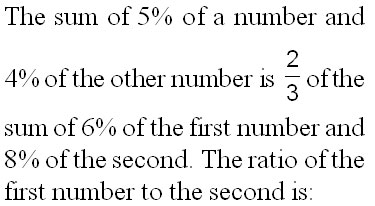(a) 2 : 3
(b) 3 : 2
(c) 3 : 4
(d) 4 : 3

40. The income of C is 20% more than B’s and the income of B is 25% more than A’s. Find by how much per cent is C’s income more than A’s?

(a) 150%
(b) 50%
(c) 25%
(d) 35%

41. In the given figure, AB || CD and EF || GH. Find the relation between a and b.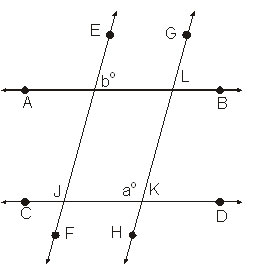(a) 2a + b=180°
(b) a + b = 180°
(c) a – b = 180°
(d) a + 2b = 180°

42. In the given figure, if l || m, find the value of x in degree.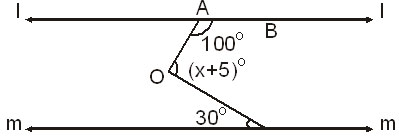(a) 105°
(b) 100°
(c) 110°
(d) 115°

43
.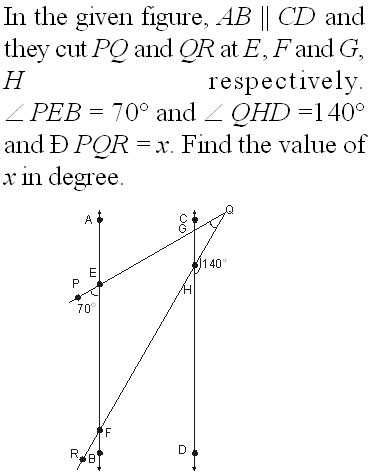(a) 20°
(b) 30°
(c) 24°
(d) 32°

44. Find the equation of the line which cuts off equal and positive intercepts on the axes and their sum is 12.

(a) x – y = 12
(b) x + y = 6
(c) x – y = 6
(d) None of these

45. The equation of the line passing through (1, 2) and parallel to 3x + 4y + 7 = 0.

(a) 3x – 4y = 11
(b) 3x + 4y =11
(c) 3x + 4y = 0
(d) None of these

46. The equation of the straight line parallel to ax + by + c = 0 and passing through the origin is

(a) ax – by = 0
(b) –bx + ay = 0
(c) ax + by = 0
(d) None of these

47. The equation of a straight line passing through (1, 2) and perpendicular to the line 3x + 4y = 7 is

(a) 4x – 3y + 2 = 0
(b) 3x + 4y = 7
(c) 4x – 3y + 7 = 0
(d) None of these

48. Which of the following is a root of the polynomial f(x) = x3 – 2×2 – x + 2?

(a) x = –2
(b) x = 1
(c) x = 3
(d) x = –3

49. If x = 2 is a root of the polynomial f (x) = 5×3 – 3×2 + kx – 10, find the value of k.

(a) – 9
(b) 3
(c) 9
(d) – 3

50. If x = 1 and –1 are the roots of the polynomial f(x) = 22 + x2 – ax + b. Find the values of a and b.

(a) 2, – 2
(b) – 2, – 1
(c) 2, – 1
(d) 1, – 2